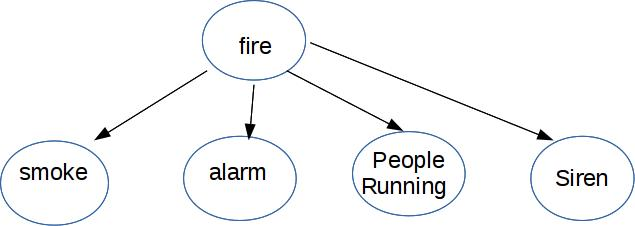CS 440/ECE 448
Margaret Fleck

## Main idea

Bayes nets (a type of Graphical Model) compactly represent how variables influence one another Here's a Bayes net with five variables.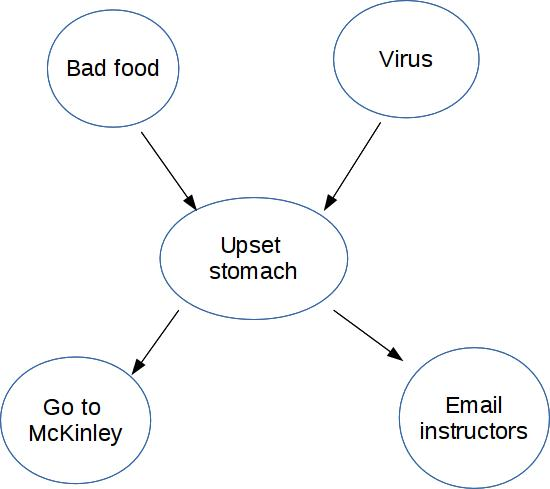For simplicity, suppose these are all boolean (T/F) variables.

Notice that this diagram shows the causal structure of the situation, i.e. which events are likely to cause which other events. For example, we know that a virus can cause an upset stomach. Technically the design of a Bayes net isn't required to reflect causal structure. But they are more compact (therefore more useful) when they do.

For each node in the diagram, we write its probability in terms of the probabilities of its parents.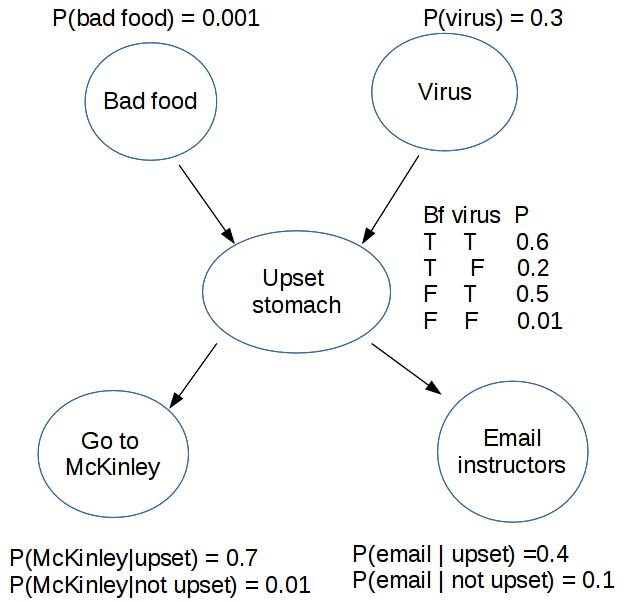"Bad food" and "Virus" don't have parents, so we give them non-conditional probabilities. "Go to McKinley" and "Email instructors" have only one parent, so we need to spell out how likely they are for both possible values of the parent node.

"Upset stomach" has two parents, i.e. two possible causes. In this case, the two causes might interact. So we need to give a probability for every possible combination of the two parent values.

## Recap: independence and conditional independence

Two random variables A and B are independent iff P(A,B) = P(A) * P(B).

A and B are conditionally independent given C iff

P(A, B | C) = P(A|C) * P(B|C)
equivalently P(A | B,C) = P(A | C)
equivalently P(B | A,C) = P(B | C)

## Conditional independence vs. independence #1

Here's a Bayes net modelling two effects with a common cause: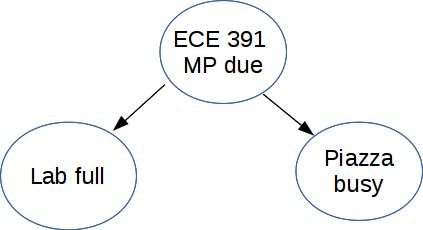Busy piazza and a full lab aren't independent, but they are conditionally independent given that there's a project due.

## Conditional independence vs. independence #2

Here's an example of two causes jointly helping create a single effect: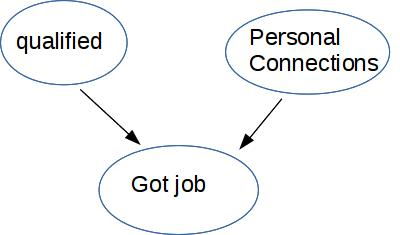Being qualified is independent of having personal connections (more or less) But they aren't conditionally independent given that the person got the job. Supppose we know that they got the job but weren't qualified, then (given this model of the causal relationships) they must have personal connections. This is called "explaining away" or "selection bias."

A high-level point: conditional independence isn't some weakened variant of independence. Neither property implies the other.

## Conditional independence vs. independence #3

Here's a causal chain, i.e. a sequence of events in which each causes the following one.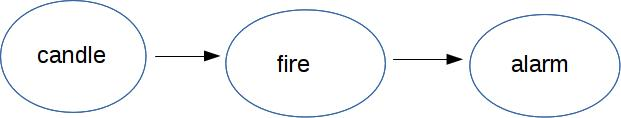Candle and alarm are conditionally independent given fire. But they aren't independent, because P(alarm | candle) is much larger than P(alarm).

## Example: independent events

A set of independent variables generates a graphical model where the nodes aren't connected.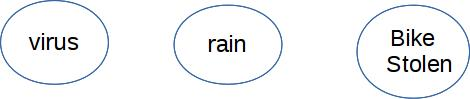## Example: Naive Bayes

Naive Bayes can be modelled with a Bayes net that has one ancestor (cause) and a number of children (effects).